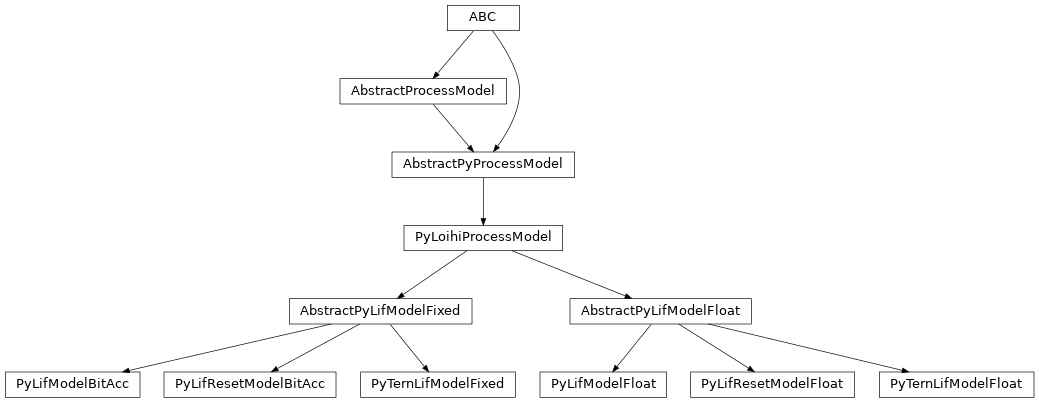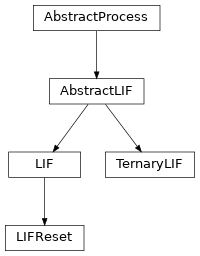# lava.proc.lif

## lava.proc.lif.modelsclass lava.proc.lif.models.AbstractPyLifModelFixed(proc_params)

Abstract implementation of fixed point precision leaky-integrate-and-fire neuron model. Implementations like those bit-accurate with Loihi hardware inherit from here.

a_in: PyInPort = LavaPyType(cls=<class 'lava.magma.core.model.py.ports.PyInPortVectorDense'>, d_type=<class 'numpy.int16'>, precision=16)
bias_exp: ndarray = LavaPyType(cls=<class 'numpy.ndarray'>, d_type=<class 'numpy.int16'>, precision=3)
bias_mant: ndarray = LavaPyType(cls=<class 'numpy.ndarray'>, d_type=<class 'numpy.int16'>, precision=13)
du: int = LavaPyType(cls=<class 'int'>, d_type=<class 'numpy.uint16'>, precision=12)
dv: int = LavaPyType(cls=<class 'int'>, d_type=<class 'numpy.uint16'>, precision=12)
reset_voltage(spike_vector)

Voltage reset behaviour. This can differ for different neuron models.

run_spk()

The run function that performs the actual computation during execution orchestrated by a PyLoihiProcessModel using the LoihiProtocol.

s_out: None
scale_bias()

Scale bias with bias exponent by taking into account sign of the exponent.

scale_threshold()

Placeholder method for scaling threshold(s).

spiking_activation()

Placeholder method to specify spiking behaviour of a LIF neuron.

subthr_dynamics(activation_in)

Common sub-threshold dynamics of current and voltage variables for all LIF models. This is where the ‘leaky integration’ happens.

u: ndarray = LavaPyType(cls=<class 'numpy.ndarray'>, d_type=<class 'numpy.int32'>, precision=24)
v: ndarray = LavaPyType(cls=<class 'numpy.ndarray'>, d_type=<class 'numpy.int32'>, precision=24)
class lava.proc.lif.models.AbstractPyLifModelFloat(proc_params=None)

Abstract implementation of floating point precision leaky-integrate-and-fire neuron model.

Specific implementations inherit from here.

a_in: PyInPort = LavaPyType(cls=<class 'lava.magma.core.model.py.ports.PyInPortVectorDense'>, d_type=<class 'float'>, precision=None)
bias_exp: ndarray = LavaPyType(cls=<class 'numpy.ndarray'>, d_type=<class 'float'>, precision=None)
bias_mant: ndarray = LavaPyType(cls=<class 'numpy.ndarray'>, d_type=<class 'float'>, precision=None)
du: float = LavaPyType(cls=<class 'float'>, d_type=<class 'float'>, precision=None)
dv: float = LavaPyType(cls=<class 'float'>, d_type=<class 'float'>, precision=None)
reset_voltage(spike_vector)

Voltage reset behaviour. This can differ for different neuron models.

run_spk()

The run function that performs the actual computation during execution orchestrated by a PyLoihiProcessModel using the LoihiProtocol.

s_out = None
spiking_activation()

Abstract method to define the activation function that determines how spikes are generated.

subthr_dynamics(activation_in)

Common sub-threshold dynamics of current and voltage variables for all LIF models. This is where the ‘leaky integration’ happens.

u: ndarray = LavaPyType(cls=<class 'numpy.ndarray'>, d_type=<class 'float'>, precision=None)
v: ndarray = LavaPyType(cls=<class 'numpy.ndarray'>, d_type=<class 'float'>, precision=None)
class lava.proc.lif.models.PyLifModelBitAcc(proc_params)

Implementation of Leaky-Integrate-and-Fire neural process bit-accurate with Loihi’s hardware LIF dynamics, which means, it mimics Loihi behaviour bit-by-bit.

Currently missing features (compared to Loihi 1 hardware):

• refractory period after spiking

• axonal delays

Precisions of state variables

• du: unsigned 12-bit integer (0 to 4095)

• dv: unsigned 12-bit integer (0 to 4095)

• bias_mant: signed 13-bit integer (-4096 to 4095). Mantissa part of neuron bias.

• bias_exp: unsigned 3-bit integer (0 to 7). Exponent part of neuron bias.

• vth: unsigned 17-bit integer (0 to 131071).

implements_process

alias of `LIF`

implements_protocol

alias of `LoihiProtocol`

required_resources: ty.List[ty.Type[AbstractResource]] = [<class 'lava.magma.core.resources.CPU'>]
s_out: PyOutPort = LavaPyType(cls=<class 'lava.magma.core.model.py.ports.PyOutPortVectorDense'>, d_type=<class 'numpy.int32'>, precision=24)
scale_threshold()

Scale threshold according to the way Loihi hardware scales it. In Loihi hardware, threshold is left-shifted by 6-bits to MSB-align it with other state variables of higher precision.

spiking_activation()

Spike when voltage exceeds threshold.

tags: ty.List[str] = ['bit_accurate_loihi', 'fixed_pt']
vth: int = LavaPyType(cls=<class 'int'>, d_type=<class 'numpy.int32'>, precision=17)
class lava.proc.lif.models.PyLifModelFloat(proc_params=None)

Implementation of Leaky-Integrate-and-Fire neural process in floating point precision. This short and simple ProcessModel can be used for quick algorithmic prototyping, without engaging with the nuances of a fixed point implementation.

implements_process

alias of `LIF`

implements_protocol

alias of `LoihiProtocol`

required_resources: ty.List[ty.Type[AbstractResource]] = [<class 'lava.magma.core.resources.CPU'>]
s_out: PyOutPort = LavaPyType(cls=<class 'lava.magma.core.model.py.ports.PyOutPortVectorDense'>, d_type=<class 'float'>, precision=None)
spiking_activation()

Spiking activation function for LIF.

tags: ty.List[str] = ['floating_pt']
vth: float = LavaPyType(cls=<class 'float'>, d_type=<class 'float'>, precision=None)
class lava.proc.lif.models.PyLifResetModelBitAcc(proc_params)

Implementation of Leaky-Integrate-and-Fire neural process with reset bit-accurate with Loihi’s hardware LIF dynamics, which means, it mimics Loihi behaviour.

Precisions of state variables

• du: unsigned 12-bit integer (0 to 4095)

• dv: unsigned 12-bit integer (0 to 4095)

• bias_mant: signed 13-bit integer (-4096 to 4095). Mantissa part of neuron bias.

• bias_exp: unsigned 3-bit integer (0 to 7). Exponent part of neuron bias.

• vth: unsigned 17-bit integer (0 to 131071).

implements_process

alias of `LIFReset`

implements_protocol

alias of `LoihiProtocol`

required_resources: ty.List[ty.Type[AbstractResource]] = [<class 'lava.magma.core.resources.CPU'>]
run_spk()

The run function that performs the actual computation during execution orchestrated by a PyLoihiProcessModel using the LoihiProtocol.

s_out: PyOutPort = LavaPyType(cls=<class 'lava.magma.core.model.py.ports.PyOutPortVectorDense'>, d_type=<class 'numpy.int32'>, precision=24)
scale_threshold()

Scale threshold according to the way Loihi hardware scales it. In Loihi hardware, threshold is left-shifted by 6-bits to MSB-align it with other state variables of higher precision.

spiking_activation()

Spike when voltage exceeds threshold.

tags: ty.List[str] = ['bit_accurate_loihi', 'fixed_pt']
vth: int = LavaPyType(cls=<class 'int'>, d_type=<class 'numpy.int32'>, precision=17)
class lava.proc.lif.models.PyLifResetModelFloat(proc_params)

Implementation of Leaky-Integrate-and-Fire neural process with reset in floating point precision.

implements_process

alias of `LIFReset`

implements_protocol

alias of `LoihiProtocol`

required_resources: ty.List[ty.Type[AbstractResource]] = [<class 'lava.magma.core.resources.CPU'>]
run_spk()

The run function that performs the actual computation during execution orchestrated by a PyLoihiProcessModel using the LoihiProtocol.

s_out: PyOutPort = LavaPyType(cls=<class 'lava.magma.core.model.py.ports.PyOutPortVectorDense'>, d_type=<class 'float'>, precision=None)
spiking_activation()

Spiking activation function for LIF.

tags: ty.List[str] = ['floating_pt']
vth: float = LavaPyType(cls=<class 'float'>, d_type=<class 'float'>, precision=None)
class lava.proc.lif.models.PyTernLifModelFixed(proc_params)

Implementation of Ternary Leaky-Integrate-and-Fire neural process with fixed point precision.

`lava.proc.lif.models.PyLifModelBitAcc`

Bit-Accurate LIF neuron model

implements_process

alias of `TernaryLIF`

implements_protocol

alias of `LoihiProtocol`

required_resources: ty.List[ty.Type[AbstractResource]] = [<class 'lava.magma.core.resources.CPU'>]
reset_voltage(spike_vector)

Reset voltage of all spiking neurons to 0.

s_out: PyOutPort = LavaPyType(cls=<class 'lava.magma.core.model.py.ports.PyOutPortVectorDense'>, d_type=<class 'int'>, precision=2)
scale_threshold()

Placeholder method for scaling threshold(s).

spiking_activation()

Placeholder method to specify spiking behaviour of a LIF neuron.

tags: ty.List[str] = ['fixed_pt']
vth_hi: ndarray = LavaPyType(cls=<class 'numpy.ndarray'>, d_type=<class 'numpy.int32'>, precision=24)
vth_lo: ndarray = LavaPyType(cls=<class 'numpy.ndarray'>, d_type=<class 'numpy.int32'>, precision=24)
class lava.proc.lif.models.PyTernLifModelFloat(proc_params=None)

Implementation of Ternary Leaky-Integrate-and-Fire neural process in floating point precision. This ProcessModel builds upon the floating point ProcessModel for LIF by adding upper and lower threshold voltages.

implements_process

alias of `TernaryLIF`

implements_protocol

alias of `LoihiProtocol`

required_resources: ty.List[ty.Type[AbstractResource]] = [<class 'lava.magma.core.resources.CPU'>]
reset_voltage(spike_vector)

Reset voltage of all spiking neurons to 0.

s_out: PyOutPort = LavaPyType(cls=<class 'lava.magma.core.model.py.ports.PyOutPortVectorDense'>, d_type=<class 'float'>, precision=2)
spiking_activation()

Spiking activation for T-LIF: -1 spikes below lower threshold, +1 spikes above upper threshold.

tags: ty.List[str] = ['floating_pt']
vth_hi: float = LavaPyType(cls=<class 'float'>, d_type=<class 'float'>, precision=None)
vth_lo: float = LavaPyType(cls=<class 'float'>, d_type=<class 'float'>, precision=None)

## lava.proc.lif.processclass lava.proc.lif.process.AbstractLIF(*, shape, u, v, du, dv, bias_mant, bias_exp, name, log_config, **kwargs)

Abstract class for variables common to all neurons with leaky integrator dynamics.

class lava.proc.lif.process.LIF(*, shape, u=0, v=0, du=0, dv=0, bias_mant=0, bias_exp=0, vth=10, name=None, log_config=None, **kwargs)

Bases: `AbstractLIF`

Leaky-Integrate-and-Fire (LIF) neural Process.

LIF dynamics abstracts to: u[t] = u[t-1] * (1-du) + a_in # neuron current v[t] = v[t-1] * (1-dv) + u[t] + bias # neuron voltage s_out = v[t] > vth # spike if threshold is exceeded v[t] = 0 # reset at spike

Parameters
• shape (tuple(int)) – Number and topology of LIF neurons.

• u (float, list, numpy.ndarray, optional) – Initial value of the neurons’ current.

• v (float, list, numpy.ndarray, optional) – Initial value of the neurons’ voltage (membrane potential).

• du (float, optional) – Inverse of decay time-constant for current decay. Currently, only a single decay can be set for the entire population of neurons.

• dv (float, optional) – Inverse of decay time-constant for voltage decay. Currently, only a single decay can be set for the entire population of neurons.

• bias_mant (float, list, numpy.ndarray, optional) – Mantissa part of neuron bias.

• bias_exp (float, list, numpy.ndarray, optional) – Exponent part of neuron bias, if needed. Mostly for fixed point implementations. Ignored for floating point implementations.

• vth (float, optional) – Neuron threshold voltage, exceeding which, the neuron will spike. Currently, only a single threshold can be set for the entire population of neurons.

Example

```>>> lif = LIF(shape=(200, 15), du=10, dv=5)
This will create 200x15 LIF neurons that all have the same current decay
of 10 and voltage decay of 5.
```
class lava.proc.lif.process.LIFReset(*, shape, u=0, v=0, du=0, dv=0, bias_mant=0, bias_exp=0, vth=10, reset_interval=1, reset_offset=0, name=None, log_config=None)

Bases: `LIF`

Leaky-Integrate-and-Fire (LIF) neural Process that resets its internal states in regular intervals.

Parameters
• shape (tuple(int)) – Number and topology of LIF neurons.

• u (float, list, numpy.ndarray, optional) – Initial value of the neurons’ current.

• v (float, list, numpy.ndarray, optional) – Initial value of the neurons’ voltage (membrane potential).

• du (float, optional) – Inverse of decay time-constant for current decay. Currently, only a single decay can be set for the entire population of neurons.

• dv (float, optional) – Inverse of decay time-constant for voltage decay. Currently, only a single decay can be set for the entire population of neurons.

• bias_mant (float, list, numpy.ndarray, optional) – Mantissa part of neuron bias.

• bias_exp (float, list, numpy.ndarray, optional) – Exponent part of neuron bias, if needed. Mostly for fixed point implementations. Ignored for floating point implementations.

• vth (float, optional) – Neuron threshold voltage, exceeding which, the neuron will spike. Currently, only a single threshold can be set for the entire population of neurons.

• reset_interval (int, optional) – The interval of neuron state reset. By default 1 timestep.

• reset_offset (int, optional) – The phase/offset of neuron reset. By defalt at 0th timestep.

`lava.proc.lif.process.LIF`

‘Regular’ leaky-integrate-and-fire neuron for

`documentation`

class lava.proc.lif.process.TernaryLIF(*, shape, u=0, v=0, du=0, dv=0, bias_mant=0, bias_exp=0, vth_hi=10, vth_lo=-10, name=None, log_config=None)

Bases: `AbstractLIF`

Leaky-Integrate-and-Fire (LIF) neural Process with ternary spiking output, i.e., +1, 0, and -1 spikes. When the voltage of a TernaryLIF neuron exceeds its upper threshold (vth_hi), it issues a positive spike and when the voltage drops below its lower threshold (vth_lo), it issues a negative spike. Between the two thresholds, the neuron follows leaky linear dynamics.

This class inherits the state variables and ports from AbstractLIF and adds two new threshold variables for upper and lower thresholds.

Parameters
• shape (tuple(int)) – Number and topology of LIF neurons.

• u (float, list, numpy.ndarray, optional) – Initial value of the neurons’ current.

• v (float, list, numpy.ndarray, optional) – Initial value of the neurons’ voltage (membrane potential).

• du (float, optional) – Inverse of decay time-constant for current decay. Currently, only a single decay can be set for the entire population of neurons.

• dv (float, optional) – Inverse of decay time-constant for voltage decay. Currently, only a single decay can be set for the entire population of neurons.

• bias_mant (float, list, numpy.ndarray, optional) – Mantissa part of neuron bias.

• bias_exp (float, list, numpy.ndarray, optional) – Exponent part of neuron bias, if needed. Mostly for fixed point implementations. Ignored for floating point implementations.

• vth_hi (float, optional) – Upper threshold voltage, exceeding which the neuron spikes +1. Currently, only a single higher threshold can be set for the entire population of neurons.

• vth_lo (float, optional) – Lower threshold voltage, below which the neuron spikes -1. Currently, only a single lower threshold can be set for the entire population of neurons.

`lava.proc.lif.process.LIF`
`documentation`